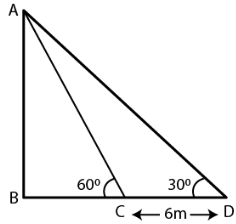Guru

# A man observes the angles of elevation of the top of a building to be 300. He walks towards it in a horizontal line through its base. On covering 60 m the angle of elevation changes to 600. Find the height of the building correct to the nearest decimal place.

• 0

sir this is the important  question from the book -ML aggarwal( avichal publication) class 10th , chapter20 , heights and distances
A man observes the angles of elevation of the top of a building to be 300.
He walks towards it in a horizontal line through its base.
On covering 60 m the angle of elevation changes to 600.

Find the height of the building correct to the nearest decimal place.

question no 20 , heights and distances , ICSE board

Share

1. It is given that

AB is a building

CD = 60 mIn triangle ABC

tan 600 = AB/BC

√3 = AB/BC

So we get

BC = AB/√3 ….. (1)

In triangle ABD

tan 300 = AB/BD

1/√3 = AB/ (BC + 60)

By cross multiplication

BC + 60 = √3 AB

BC = √3 AB – 60

Using both the equations we get

AB/√3 = √3 AB – 60

By further calculation

AB = 3AB – 60√3

3AB – AB = 60 × 1.732

So we get

AB = (60 × 1.732)/ 2

AB = 51.96 m

• 0